## TILTSHIFT

 Applies a tiltshift effect to an image

 USAGE: tiltshift [-m mode] [-s size] [-b blur] [-B brightness] [-C contast] [-S saturation] infile outfile USAGE: tiltshift [-help|-h] -m ... mode ......... mode of tiltshift; choices are: vertical (v), ..................... horizontal (h), elliptical (e) and circular (c); ..................... default=vertical -s ... size ......... size of central unblurred area; -100<=integer<=100; ..................... default=0 (no change from default amount) -b ... blur ......... blur amount for outer area; float>=0; default=5 -B ... brightness ... brightness change of image; -100<=integer<=100; ..................... default=0 (no change) -C ... contrast ..... contrast change of image; -100<=integer<=100; ..................... default=0 (no change) -S ... saturation ... saturation change of image; -100<=integer<=100; ..................... default=0 (no change) PURPOSE: Applies a tiltshift effect to an image. DESCRIPTION: TILTSHIFT applies a tiltshift effect to an image. There are optional modes including: vertical, horizontal, elliptical and circular ARGUMENTS: -m mode ... MODE of the tiltshift effect. The choices are: vertical (v), horizontal (h), elliptical (e) and circular (c). The default=vertical. -s size ... SIZE of the central unblurred area relative to a default amount. Values are -100<=integers<=100. The default=0. -b blur ... BLUR amount for the outer area. Values are floats>=0. The default=5. -B brightness ... BRIGHTNESS change of the image. Values are -100<=integers<=100. The default=0 (no change). -C contrast ... CONTRAST change of the image. Values are -100<=integers<=100. The default=0 (no change). -S saturation ... SATURATION change of the image. Values are -100<=integers<=100. The default=0 (no change). CAVEAT: No guarantee that this script will work on all platforms, nor that trapping of inconsistent parameters is complete and foolproof. Use At Your Own Risk.

### EXAMPLES

 Example 1 -- Vertical Mode -- Variation in Size (Taken by Anthony Thyssen) Original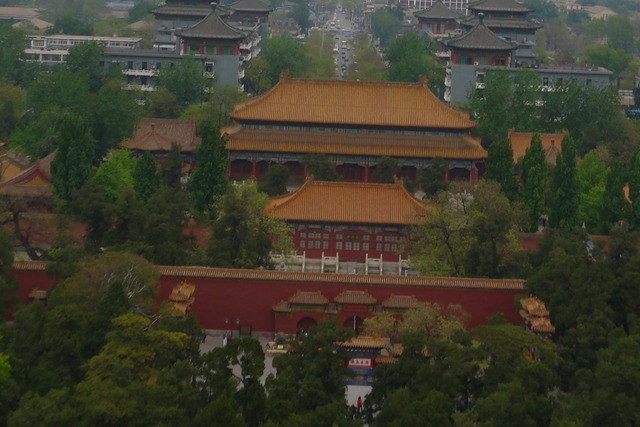Arguments: -m vertical -s -25 -B 50 -C 20Arguments: -m vertical -s 0 -B 50 -C 20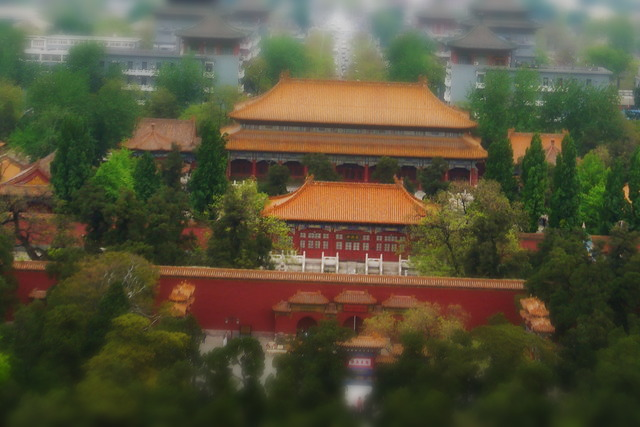Arguments: -m vertical -s 75 -B 50 -C 20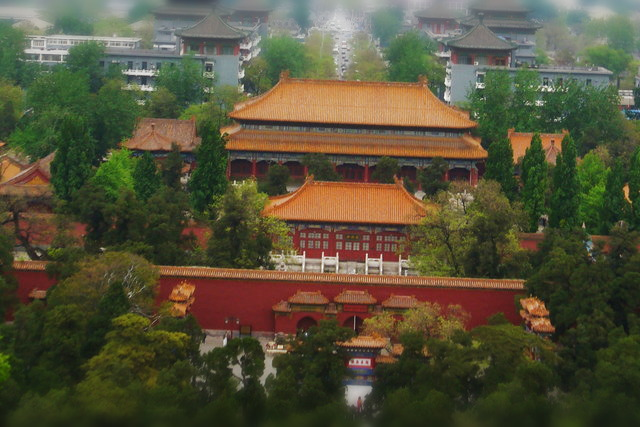Example 2 -- Horizontal Mode -- Variation in Size (Taken by Anthony Thyssen) OriginalArguments: -m horizontal -s -25 -B 50 -C 20Arguments: -m horizontal -s 0 -B 50 -C 20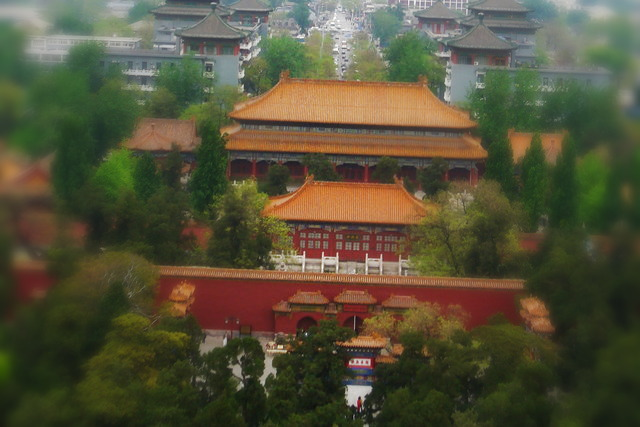Arguments: -m horizontal -s 75 -B 50 -C 20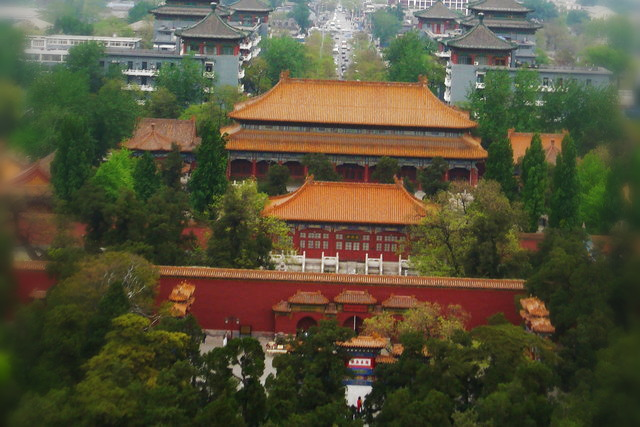Example 3 -- Elliptical Mode -- Variation in Size (Taken by Anthony Thyssen) OriginalArguments: -m ellipse -s -25 -B 50 -C 20Arguments: -m ellipse -s 0 -B 50 -C 20Arguments: -m ellipse -s 75 -B 50 -C 20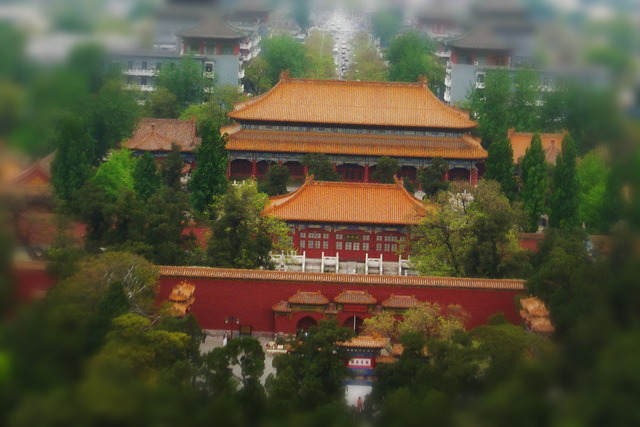Example 4 -- Circular Mode -- Variation in Size (Taken by Anthony Thyssen) OriginalArguments: -m circle -s -25 -B 50 -C 20Arguments: -m circle -s 0 -B 50 -C 20Arguments: -m circle -s 75 -B 50 -C 20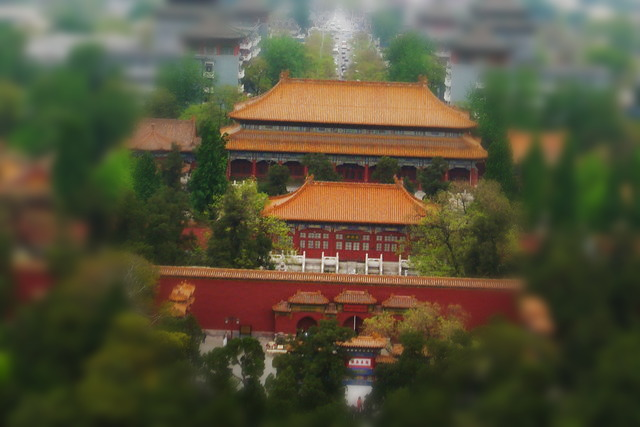What the script does is as follows: Creates either a vertical or horisontal gradient that is solarize to reflect it or an elliptical or circular gradient to be used as a mask Enhances the image in brightness, contrast and/or saturation Blurs the enhanced image Uses the gradient mask to composite the two previous images This is equivalent to the following IM commands for the case of mode=vertical: convert -quiet -regard-warnings "\$infile" +repage "\$tmpA1" ww=`identify -ping -format "%w" \$tmpA1` hh=`identify -ping -format "%h" \$tmpA1` dim=`convert xc: -format "%[fx:min(\$ww,\$hh)]" info:` convert -size \${ww}x\${hh} gradient: -solarize 50% -level 0x50% -negate \$tmpA2 bright=`convert xc: -format "%[fx:\$bright+100]" info:` sat=`convert xc: -format "%[fx:\$sat+100]" info:` contr=`convert xc: -format "%[fx:(2/10)*abs(\$contr)]" info:` test=`convert xc: -format "%[fx:abs(\$contr)<0.0001?0:(\$contr>0?1:-1)]" info:` if [ \$test -eq 1 -o \$test -eq 0 ]; then sign="-" elif [ \$test -eq -1 ]; then sign="+" fi test=`convert xc: -format "%[fx:\$size<1?0:1]" info:` if [ \$test -eq 1 ]; then size=`convert xc: -format "%[fx:-0.0099*\$size+1]" info:` elif [ \$test -eq 0 ]; then size=`convert xc: -format "%[fx:-0.09*\$size+1]" info:` fi convert \( \$tmpA1 -modulate \$bright,\$sat,100 \${sign}sigmoidal-contrast \${contr},50% \) \ \( -clone 0 -blur 0x\$blur \) \ \( \$tmpA2 -gamma \$size \) \ \$setcspace -compose over -composite \$outfile